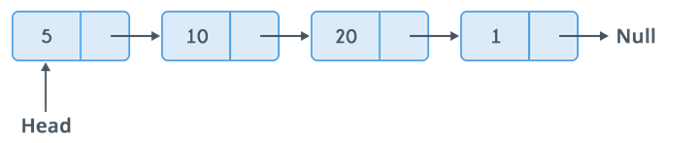# Single Linked list and its basic operations with traversing implementation

The article describes the single linked list ADT and its traversal implementation.
Submitted by Radib Kar, on October 21, 2018

Single linked list contains a number of nodes where each node has a data field and a pointer to next node. The link of the last node is to NULL, indicates end of list.The node in the linked list can be created using struct.

```struct node{
// data field, can be of various type, here integer
int data;
// pointer to next node
struct node* next;
};
```

### Basic operations on linked list

• Traversing
• Inserting node
• Deleting node

### Traversing technique for a single linked list

• Display content of current nodes
• Stop when next pointer is NULL

### C code to traverse a linked list and count number of nodes

```#include <stdio.h>
#include <stdlib.h>

struct node{
int data; // data field
struct node *next;
};

int count=0; // to count total no of nodes
printf("\n traversing the list\n");

//traverse until current node isn't NULL
while(current!=NULL){
count++; //increase node count
printf("%d ",current->data);
current=current->next; // go to next node
}
printf("\ntotal no of nodes : %d\n",count);
}

struct node* creatnode(int d){
struct node* temp= (struct node*) malloc(sizeof(struct node));
temp->data=d;
temp->next=NULL;
return temp;
}

int main()
{

//same linked list is built according to image shown

return 0;
}
```

Output

```creating & traversing linked list

traversing the list
5 10 20 1
total no of nodes : 4
```

Time complexity:

O(n), for scanning the list of size n

Space complexity: